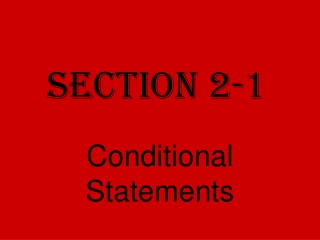Download PresentationSection 2-1

# Section 2-1 - PowerPoint PPT PresentationDownload Presentation## Section 2-1

- - - - - - - - - - - - - - - - - - - - - - - - - - - E N D - - - - - - - - - - - - - - - - - - - - - - - - - - -
##### Presentation Transcript

1. Section 2-1 Conditional Statements

2. Conditional statements • Have two parts: • Hypothesis (p) • Conclusion (q)

3. "If p, then q:” p q When written in If-Then form… • The “If” part ALWAYS contains the hypothesis • The “Then” part ALWAYS contains the conclusion

4. Example #1 • If it is a Friday, then you will not have homework. it is a Friday Hypothesis (p): Conclusion (q): you will not have homework

5. Do not include the words “If” and “then” when naming the hypothesis and conclusion!

6. Sometimes a conditional statement is written without using "if" and "then".

7. Example #2 • Perpendicular lines intersect at right angles. Can rewrite: If two lines are perpendicular, then the lines intersect at right angles.

8. If two lines are perpendicular, then the lines intersect at right angles. Hypothesis (p): two lines are perpendicular Conclusion (q): the lines intersect at right angles

9. Conditional statements can be true or false.

10. counterexample • an example that disproves a statement • Only need one counterexample to disprove a statement

11. Example #3 • If a number is odd, then it is divisible by 3. False Counterexample: 5 is odd and is not divisible by 3!

12. Conditional : p q Converse : q p converse • formed by interchanging the hypothesis and conclusion of the conditional.

13. Example #4 • If two lines are perpendicular, then the lines intersect at right angles. Write the converse. If two lines intersect at right angles, then the lines are perpendicular.

14. inverse • the negation of both the hypothesis and the conclusion of the conditional. • The denial of a statement is called a negation.

15. Conditional:p q ~ p ~ q Inverse: Read as not p then not q

16. Example #5 • If two lines are perpendicular, then the lines intersect at right angles. If two lines are not perpendicular, then the lines do not intersect at right angles. Inverse:

17. Converse: q p ~q ~p contrapositive • negation of both the hypothesis and conclusion of the converse Contrapositive: Read as not q then not p

18. Example #6 • If two lines are perpendicular, then the lines intersect at right angles. Write the converse. If two lines intersect at right angles, then the lines are perpendicular.

19. Then write the contrapositive. If two lines do not intersect at right angles, then the lines are not perpendicular.

20. Equivalent statements • Statements that are both true or both false

21. A conditional statement is equivalent to its contrapositive Conditional = Contrapositive • The converse and inverse of any conditional statement are equivalent. Converse = Inverse